## NPN Characteristic Curves#### Measured Characteristic Curves for 2N3904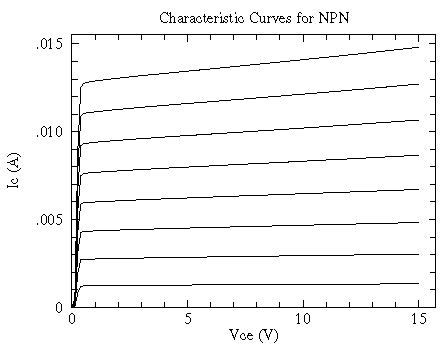for IB = 10 µA, 20 µA, 30 µA, ... , 80 µA

big data file, smaller data file, postscript plot, pdf plot

The behavior of an NPN bipolar transistor is largely controlled by the current flowing into the base (i.e., a positive current). For the usual collector-emitter voltage drops (i.e., the active region: positive voltages from a fraction of a volt up to some breakdown voltage) the collector current (IC) is nearly independent of the collector-emitter voltage (VCE), and instead depends on the base current (IB). (This is unusual behavior: usually more voltage produces to more current, but here the current only increases slightly with increasing VCE.) The current gain, i.e., the ratio of the collector current to the base current, is often denoted by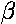or hFE:= hFE = IC/IB

Thus in the simplest approximation the characteristic curves of a NPN are a set of flat, evenly spaced, lines: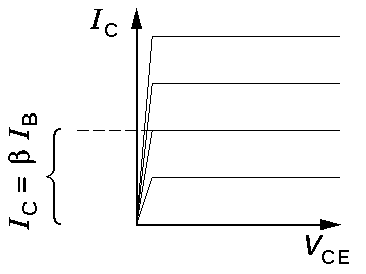Each (flat) curve shows that IC doesn't change with changing VCE. The different levels show that IC does depend on IB.

A slightly more complicated approximation takes into account the sloping characteristic curves through the fiction of a constant Early Voltage (VA). Here we assume that the characteristic curves all have a common x-axis intercept at the large negative voltage -VA. (The dashed curves are far from the active region and in no way represent the actual behavior of the transistor for negative VCE. In fact, the transistor is not designed to be operated with negative VCE.)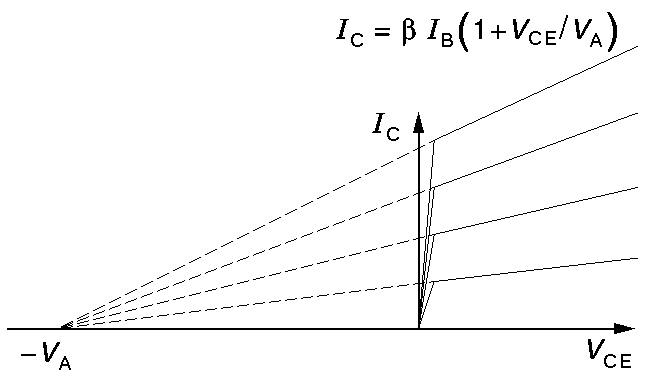(For the above measured 2N3904, the Early voltage ranges from 100 to 150 V. That is the extrapolated characteristic curves do not intersect at a point.)

The actual relationship between the collector current (IC) and the controlling base current (IB) and collector-emitter voltage drop (VCE) is some complicated function which we can denote:

IC(IB,VCE)

Like any function we can approximate it near a particular point using just the first terms of a Taylors expansion: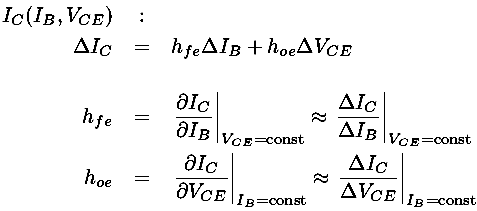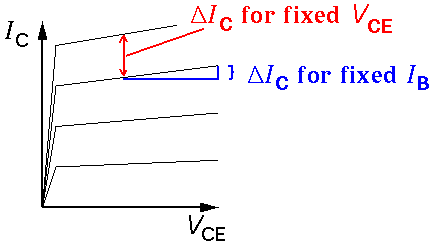Clearly these hybrid (h) parameters are not constants. For example hoe is the slope of a characteristic curve, which is nearly zero for small IB and increases for larger IB. (Note that slope on an I-V is basically the inverse of the resistance. Thus 1/hoe can be described as the output impedance. A typical value for 1/hoe would be 100,000.)

Even the defining parameter -- the current gainor hFE -- is not particularly constant and depends on IB. Here is the measured relationship for the above 2N3904: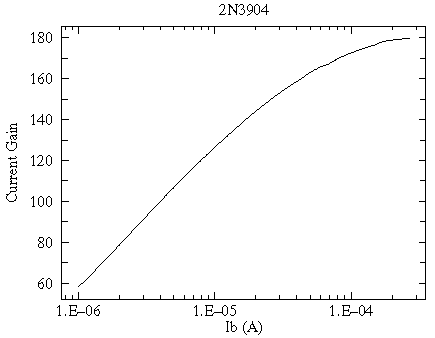The characteristic curves focus on the output of the transistor, but we can also consider the behavior of the input. In the active region the base is a forward biased diode, and so VB would be about .7 V, typical for a conducting Si diode. Of course in greater detail the relationship between VB and IB would be given by the Shockley diode equation: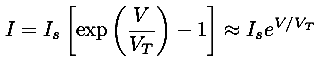where Is is a constant and the thermal voltage VT is given by:Because of the exponential relationship between base current and base voltage, the slope of this relationship (which could be called the input conductance, or 1/rB, or 1/hie) can be approximated by: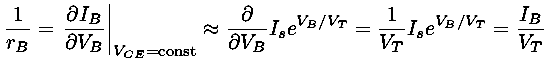where in the last equation (25/IB) the base current must be entered in mA.

A desirable characteristic of a transistor is that the outputs have little effect on the inputs, but if we look in detail we find that VCE affects VB. The actual functional relationship giving the base voltage (VB) from the base current (IB) and collector-emitter voltage drop (VCE) is some complicated function which we can denote:

VB(IB,VCE)

Like any function we can again approximate it near a particular point using just the first terms of Taylors expansion: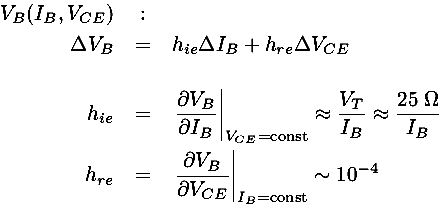The small value of hre shows that the input is largely unaffected by the output.

The spec sheet reports the following values for the 2N3904:

Characteristics Symbol Min Max Unit
Input Impedance hie 1 10 k Ohms
Voltage Feedback Ratio hre 0.5 8 ×10-4
Small-Signal Current Gain hfe 100 400
Output Admittance hoe 1.0 40 µ mhos